# SETS - SOME IMPORTANT NUMBER SETS

SETS - SOME IMPORTANT NUMBER SETS

SET

A set is a collection of well defined objects which are distinct from each other

Set are generally denoted by capital letters A, B, C, .... etc. and the elements of the set by a, b, c .... etc.
If a is an element of a set A, then we write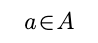and say a belongs to A.
If a does not belong to A then we write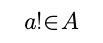e.g. The collection of first five prime natural numbers is a set containing the elements 2, 3, 5, 7, 11.

SOME IMPORTANT NUMBER SETS :

N = Set of all natural numbers = {1, 2, 3, 4, ....}

W = Set of all whole numbers = {0, 1, 2, 3, ....}

Z or I set of all integers    = {.... –3, –2, –1, 0, 1, 2, 3, ....}

Z+  =  Set of all +ve integers = {1, 2, 3, ....} = N.
Z–  =  Set of all –ve integers = (–1, –2, –3, ....}
Z0 =   The set of all non-zero integers. = {±1, ±2, ±3, ....}
Q =    The set of all rational numbers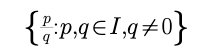R = the set of all real numbers.

R–Q = The set of all irrational numbers

e.g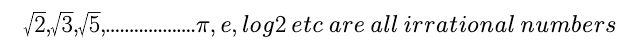METHODS TO WRITE A SET :

(i) Roster Method : In this method a set is described by listing elements, separated by commas and enclose then by curly brackets
e.g. The set of vowels of English Alphabet may be described as {a, e, i, o, u}

(ii) Set Builder Form : In this case we write down a property or rule p Which gives us all the element
of the set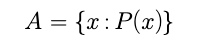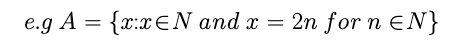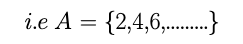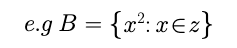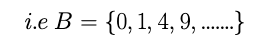TYPES OF SETS

Null set of Empty set :

A set having no element in it is called an Empty set or a null set or void set it is denoted by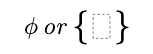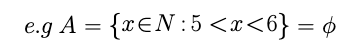A set consisting of at least one element is called a non - empty set or non - void set.

Example :

The set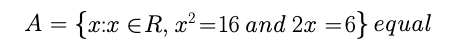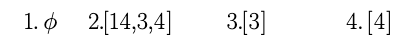Solution :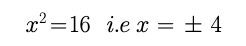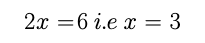There is no value of x which satisfies both the above equations

Thus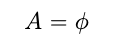Singleton :

A set consisting of a single element is called a singleton set. e.g. Then set {0}, is a singleton set.

Finite Set :

A set which has only finite number of elements is called a finite set. e.g. A = {a, b, c}

Order of a finite set :

The number of elements in a finite set is called the order of the set A and is denoted O(A) or n(A). It is also called cardinal number of the set.

e.g.A={a,b,c,d}  i.e n(A)=4

Infinite set :

A set which has an infinite number of elements is called an infinite set.

e.g. A = {1, 2, 3, 4, ....} is an infinite set

Equal sets :

Two sets A and B are said to be equal if every element of A is a member of B, and every element of B is a member of A.

If sets A and B are equal. We write A = B and A and B are not equal then A != B

e.g.A={1,2,6,7} and B={6,1,2,7} i.e A=B

Equivalent sets :

Two finite sets A and B are equivalent if their number of elements are same ie. n(A) = n(B)

e.g.A={1,3,5,7}, B={a,b,c,d}

n(A) = 4 and n(B) = 4 i.e n(A) = n(B)

Note : Equal set always equivalent but equivalent sets may not be equal
Subsets :

Let A and B be two sets if every element of A is an element B, then A is called a subset of B if A is a subset of B. we write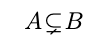Example : A={1,2,3,4}andB={1,2,3,4,5,6,7}

i.eProper subset :

If A is a subset of B and A != B then A is a proper subset of B. and we write

Note-1 : Every set is a subset of itself i.e. A A for all A
Note-2 : Empty set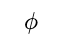is a subset of every set

Note-3 : The total number of subsets of a finite set containing n elements is 2n

Universal set : A set consisting of all possible elements which occur in the discussion is called a Universal set and is denoted by U

Note : All sets are contained in the universal set

e.g. If A = {1, 2, 3}, B = {2, 4, 5, 6}, C = {1, 3, 5, 7} then U = {1, 2, 3, 4, 5, 6, 7} can be taken as the Universal set.
Power set : Let A be any set. The set of all subsets of A is called power set of A and is denoted by P(A)

Power set : Let A be any set. The set of all subsets of A is called power set of A and is denoted by P(A)

Let A = {1, 2}. then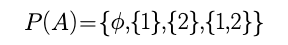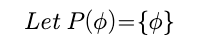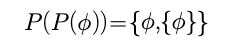then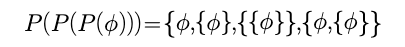Note-1 : Ifthen P(A) has one element

Note-2 : Power set of a given set is always non empty

Example :

Two finite sets of have m and n elements respectively the total number of elements in power set of first set is
56 more thatn the total number of elements in power set of the second set find the value of m and n
respectively.

Solution :

Number of elements in power set of 1st set =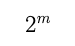Number of elements in power set of 2nd set =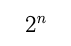Given,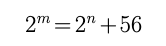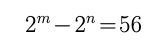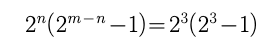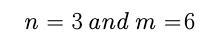Post By : Rahul Kumar 16 May, 2020 519 views Maths